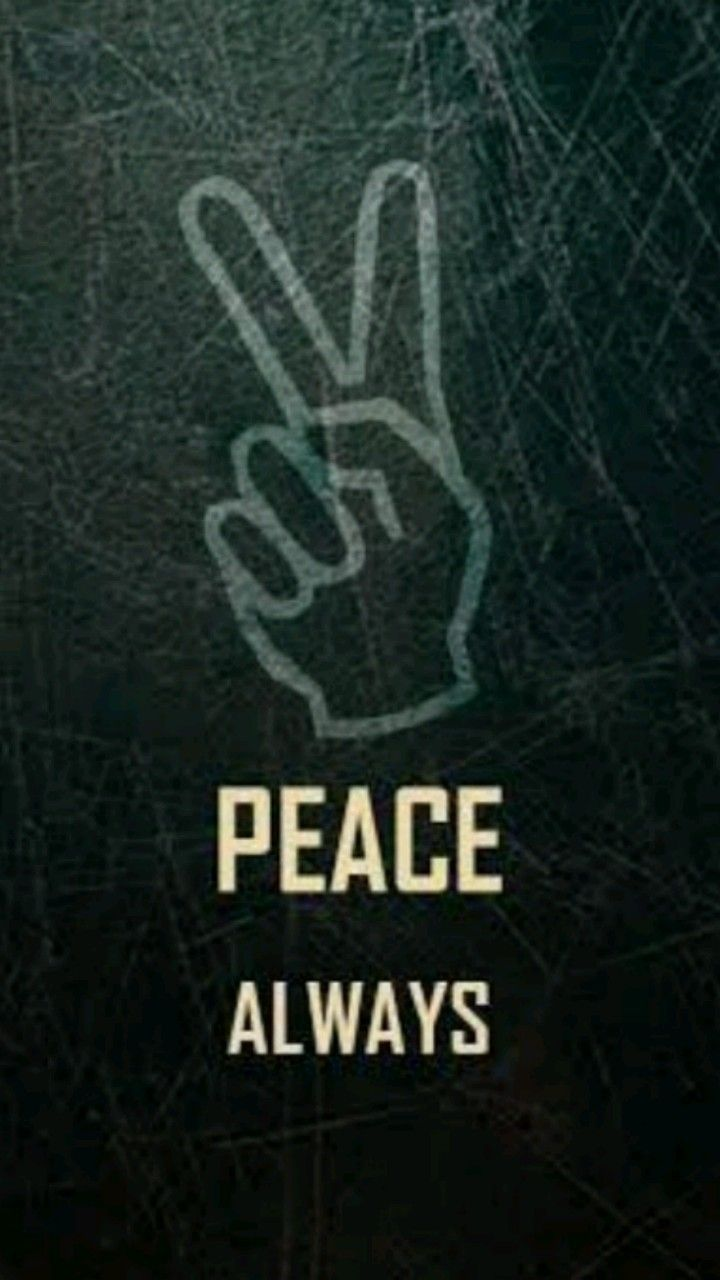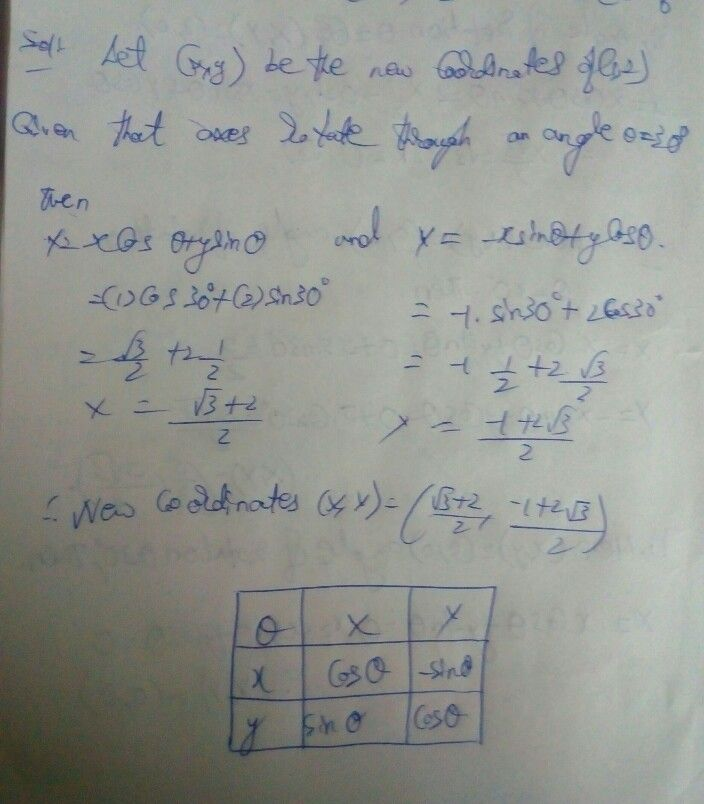Symbol
ProblemIt the axes $2$ are rotated through $\square$ angle $3y2$ then find the coordinates of $\left(1,2\right)$ $0$ the $=$ $52-\bar{=}$ $4$ $x$ $74\right)$
Other
Search count: 108
SolutionQanda teacher - aakarcheck the answer plseStudent
thanks